Ch 12. Infinite Sequences and Series Multimedia Engineering Math Sequencesand Series Integral andComparison Alt. Series &Abs. Conv. PowerSeries Taylor, Mac. &Binomial
 Chapter 1. Limits 2. Derivatives I 3. Derivatives II 4. Mean Value 5. Curve Sketching 6. Integrals 7. Inverse Functions 8. Integration Tech. 9. Integrate App. 10. Parametric Eqs. 11. Polar Coord. 12. Series Appendix Basic Math Units Search eBooks Dynamics Fluids Math Mechanics Statics Thermodynamics Author(s): Hengzhong Wen Chean Chin Ngo Meirong Huang Kurt Gramoll ©Kurt GramollMATHEMATICS - THEORY The series introduced thus far have been those with non-negative terms. In this section, series with mixed signs such as the alternating series will be introduced. Several convergence testing methods, such as the alternating series test, ratio test and root test, will be presented. Alternating Series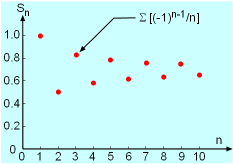The Plot of the Partial Sum for an Alternate Harmonic Series Σ[(-1)n-1/n] as a Function of n. As the name of the alternate series imply, these series have consecutive terms with alternate signs. An example of alternating series is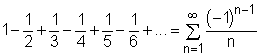The above series is also known as the alternating harmonic series. Note that the last term of the series has the form an = (-1)n-1bn where bn is positive. The plot of the partial sum as a function of n is shown in the figure on the left. Alternating Series Test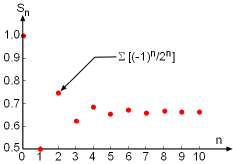Plot of the Partial Sum for an Alternate Series Σ[(-1)n/2n] as a Function of n. According to the alternating series test, when a series consists of (a) terms with alternate signs, (b) terms which decreases in magnitude      (i.e.,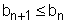for all n), and (c) the nth term (an) has limit of zero      (i.e.,), then the series converges. Take the series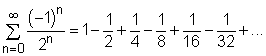for example. Obviously, this series has alternating terms. The absolute magnitude of the terms decrease as n increases (i.e.,). And as n tends to infinity, nth term has a limit of zero. Hence, by alternating series test, this series converges. Often partial sum Sn is used as an estimation to the total sum S of a convergent series. How many terms are required to provide a reasonable good estimation? What is the error involved? For a series which satisfies the alternating series test, the remainder of using Sn as an estimation of S is given by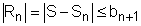Absolute Convergence Test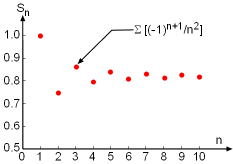Plot of the Partial Sum for an Alternate Series Σ[(-1)n+1/n2] as a Function of n. A series Σan is said to converge absolutely if the series of absolute terms (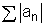) converges. A series is convergent if it is absolute convergent. However, a series is conditionally convergent if it is convergent but not absolutely convergent. For example, the series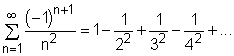is absolute convergent since it was proved previously, using the basic comparison and integral tests, that the following series (p-series with p = 2)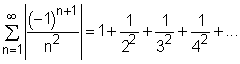converges.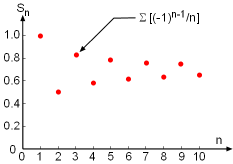The Plot of the Partial Sum for an Alternate Harmonic Series Σ[(-1)n-1/n] as a Function of n. On the other hand, the alternating harmonic series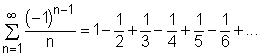only converges conditionally. The above series is convergent but the series with absolute terms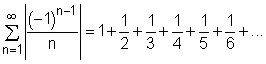diverges. Ratio Test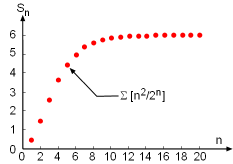Plot of the Partial Sum for a Series Σ[n2/2n] as a Function of n. Another useful test in examining whether a series is convergent or divergent is the ratio test, which is stated as follows: (1) The series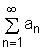is absolutely convergent if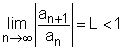(2) The seriesis divergent if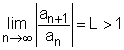Note that if the limit tends to 1 then the ratio test gives no information regarding the convergency. The ratio test is particularly useful when examining series with terms that consist of factorials and nth powers of constants. Take the series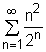for example. According to the ratio test: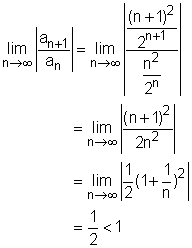Hence, this series is absolutely convergent. Root Test Besides the ratio test, the root test can also be used to examine if a series is convergent or divergent. The root test is convenient in determining series with terms raised to the nth power, and it is stated as follows: (1) The seriesis absolutely convergent if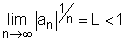(2) The seriesis divergent if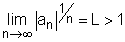The serieswas shown to be absolute convergent using the ratio test. Using the root test: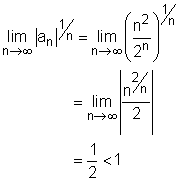it is also determined that the series is absolute convergent.